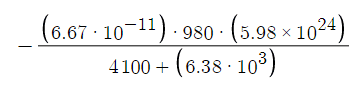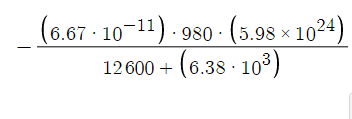# Given two Earth satellites, A and B, find the potential energy/kinetic energy

MiSTSiM
Homework Statement:
Two Earth satellites, A and B, each of mass m = 980 kg , are launched into circular orbits around the Earth's center. Satellite A orbits at an altitude of 4100 km , and satellite B orbits at an altitude of 12600 km .

Part A
What are the potential energies of the two satellites?

Part B
What are the kinetic energies of the two satellites?

Part C
How much work would it require to change the orbit of satellite A to match that of satellite B?
Relevant Equations:
-G*M_e*m/r

r= km from center of earth which is the altitude + radius of earth

Change in U + Change in K=0= -3.7298538168*10^13, -2.0594767123*10^13

Ive tried this equation with both masses and my homework keeps coming back with the wrong answer. I've tried checking my arithmetic but I cannot find anything wrong with it please help​
haven't gotten the chance to try B but I am pretty sure it will be the same as A but positive is that right?​

Homework Helper
Gold Member
Homework Statement:: Two Earth satellites, A and B, each of mass m = 980 kg , are launched into circular orbits around the Earth's center. Satellite A orbits at an altitude of 4100 km , and satellite B orbits at an altitude of 12600 km .

Part A
What are the potential energies of the two satellites?

Part B
What are the potential energies of the two satellites?

Part C
How much work would it require to change the orbit of satellite A to match that of satellite B?
Relevant Equations:: -G*M_e*m/r

How does the question in Part B differ from part A?

The denominators should be in meters not kilometers.

•Electrodynamics_ and Delta2
MiSTSiM
How does the question in Part B differ from part A?

The denominators should be in meters not kilometers.
Thank you that came back correct and part B was meant to say kinetic energy I copied it from the first just forgot to change that part to kinetic.

Homework Helper
2022 Award

•Steve4Physics, PeroK and Orodruin
Staff Emeritus
Homework Helper
Gold Member
The denominators should be in meters not kilometers.
”Should be” is a bit strong, g m^2/(s^2) is a valid energy unit after all - although not the typical SI unit. What is really missing is any units at all and the underlying assumption that everything is in the basic SI units when inserting a length in km.

Homework Helper
Gold Member
”Should be” is a bit strong, g m^2/(s^2) is a valid energy unit after all - although not the typical SI unit. What is really missing is any units at all and the underlying assumption that everything is in the basic SI units when inserting a length in km.
I agree that the the missing units are a real problem. Perhaps if the OP had considered units, the numerical answer would have been correct. However, I think that "should be" is appropriately strong. Apparently, this question was scored by an algorithm that is not artificially intelligent enough to recognize numerical answers in units other than SI.

Staff Emeritus
Homework Helper
Gold Member
I agree that the the missing units are a real problem. Perhaps if the OP had considered units, the numerical answer would have been correct. However, I think that "should be" is appropriately strong. Apparently, this question was scored by an algorithm that is not artificially intelligent enough to recognize numerical answers in units other than SI.
I guess it all depends on how the question was asked. If the question asked for an answer in units of Joule then there is of course only one correct measured value. (Some web forms and similar will give a textbox in which to enter a number and then a unit after it for example.)

Homework Helper
2022 Award
In some courses I would deduct (additional) points for careless units and wildly inappropriate Sig Fig such that no response garnered a higher score than a grotesquely inappropriate one.

•Mayhem
Mayhem
In some courses I would deduct (additional) points for careless units and wildly inappropriate Sig Fig such that no response garnered a higher score than a grotesquely inappropriate one.
Lack of units is a much more severe offense than sig figs imo. No information is lost in forgetting to round, but without units I don't know if it's km, feet or inches (in principle).

Staff Emeritus
Homework Helper
Gold Member
No information is lost in forgetting to round
While I agree that skipping the units is worse. It is also not ok to artificially give the impression to create information that simply isn’t there. I have several examples of this going wrong.

•PeroK
Homework Helper
2022 Award
While I agree that skipping the units is worse.

Both hanging offenses but equally bad IMO, at least for engineers. My least favorite answer was nine sig fig but off by 10^12. A slide rule was good training for me.

Homework Helper
Gold Member
In my book, messing up the units is worse and can cause palpable harm. In 1999 NASA lost its \$125-million Mars Climate Orbiter because spacecraft engineers failed to convert from English to metric measurements when exchanging vital data before the craft was launched. I remember when it happened and guess what I told my intro physics class after it did?

Here is the LA Times story.

•hutchphd and PeroK
Staff Emeritus